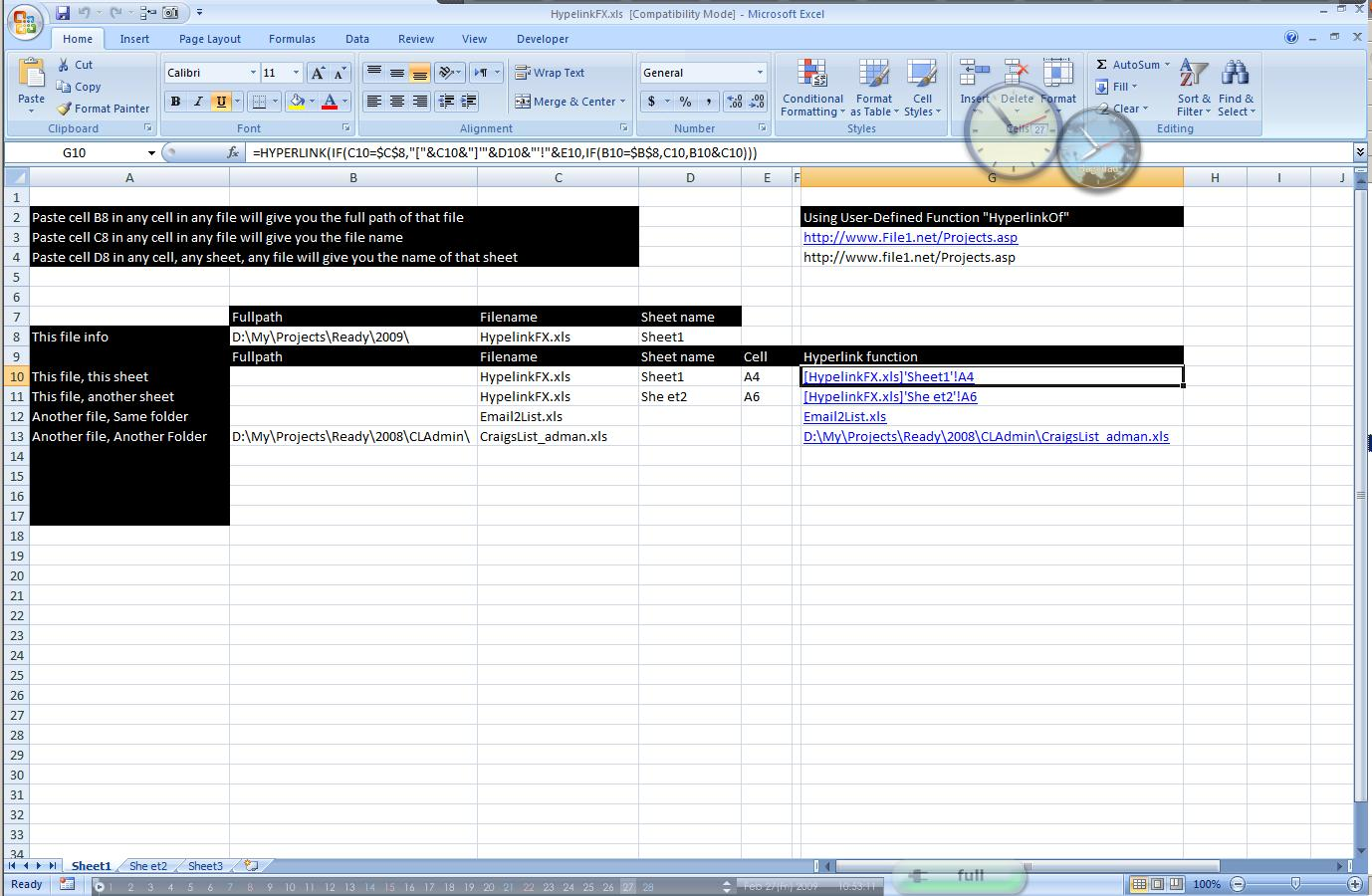The Hyperlink function is really powerful and yet not widely used.

Of course the “Jump-to” link need other functions, like Match, Indirect and Index

Here is a sample of what we can do with Hyperlink and other functions

This one has a lot of formulas to make it work well:

First: we have the formula that brings the path where this Excel file is saved:

`=LEFT(CELL("filename",\$A\$1),SEARCH("[",CELL("filename",\$A\$1))-1)`

Then: we have the one that grabs This Excel file name:

`=MID(CELL("filename",\$A\$1),SEARCH("[",CELL("filename",\$A\$1))+1,SEARCH("]",CELL("filename",\$A\$1),SEARCH("[",CELL("filename",\$A\$1)))-SEARCH("[",CELL("filename",\$A\$1))-1)`

Then: The one that brings Active sheet name:

`=MID(CELL("filename",\$A\$1),SEARCH("]",CELL("filename",\$A\$1))+1,500)`

And off course: the one that Constructs the Hyperlink itself

`=HYPERLINK(IF(C10=\$C\$8,"["&C10&"]'"&D10&"'!"&E10,IF(B10=\$B\$8,C10,B10&C10)))`

And as a bonus, the UDF that reads the URL from a flat Hyperlink:

`=hyperlinkof(G3)`